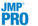Predictive and Specialized Modeling > K Nearest Neighbors > The K Nearest Neighbors Report
Publication date: 07/30/2020

#The K Nearest Neighbors Report

The K Nearest Neighbors report contains a separate report for each response variable. Each response variable report contains information about the fitted model for that response. This information includes a Model Selection report and summary information for each of the k models that were fit. The report shows tables for the training set and for the validation and test sets if you defined these using validation.

The Model Selection report displays a solution path plot across K based on the Misclassification Rate for categorical responses or the RMSE for continuous responses. By default, the slider is placed on the value of K that corresponds to the best performing model. You can drag the slider to change the value of K in the report.

The statistics reported depend on the modeling type of the response. Each row in the summary tables corresponds to a model defined by k nearest neighbors, where K ranges from one to the value that you specified as Number of Neighbors, K in the launch window.

##Continuous Responses

By default, in addition to the Model Selection graph, the report for a continuous response contains the Summary Table report.

#### Summary Table

An asterisk marks the model for the value of K that has the smallest RMSE. The report for a continuous response contains the following columns:

K

Number of nearest neighbors used in the model. K ranges from 1 to the Number of Neighbors, K that you specified in the launch window.

Count

Number of observations.

RSquare

The RSquare value for the model.

RMSE

Root mean square error for the model. The model with the smallest RMSE is marked with an asterisk. If there are tied RMSE values, the model with the smallest K is marked with the asterisk.

SSE

Sum of squared errors for the model.

##Categorical Responses

By default, in addition to the Model Selection graph, the report for a categorical response contains the Summary Table, Confusion Matrix, and Mosaic Plot reports.

#### Summary Table

An asterisk marks the model for the value of K that has the smallest misclassification rate. The report for a categorical response contains the following columns:

K

Number of nearest neighbors used in the model. K ranges from 1 to the Number of Neighbors, K that you specified in the launch window.

Count

Number of observations.

Misclassification Rate

Proportion of observations misclassified by the model. This is calculated as Misclassifications divided by Count. The model with the smallest misclassification rate is marked with an asterisk. If there are tied misclassification rates, the model with the smallest K is marked with the asterisk.

Misclassifications

Number of observations that are incorrectly predicted by the model.

#### Confusion Matrix

By default, a confusion matrix is shown for the model with the smallest Misclassification Rate. If there are ties for the smallest misclassification rate, a confusion matrix is shown for the model with the smallest K. If you use validation, confusion matrices for the validation and test sets appear. A confusion matrix is a two-way classification of actual and predicted responses. Use the confusion matrices and the misclassification rates to evaluate your model.

Tip: If you change the position of the slider in the solution path plot, an additional Confusion Matrix is displayed for the chosen value of K. Use the additional confusion matrices to compare an alternative model to the default best model.

#### Mosaic Plot

By default, a mosaic plot is shown for the model with the smallest Misclassification Rate. If there are ties for the smallest misclassification rate, a mosaic plot is shown for the model with the smallest K. A mosaic plot is a stacked bar chart where each segment is proportional to its group’s frequency count. For more information about mosaic plots, see Mosaic Plot in Basic Analysis. If you use validation, mosaic plots for the validation and test sets are shown.

Tip: If you change the position of the slider in the solution path plot, the mosaic plot updates to display the results for the chosen value of K.